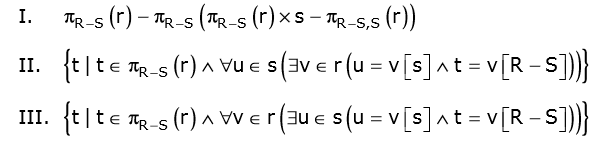Related Articles
GATE | GATE-CS-2009 | Question 60
• Difficulty Level : Expert
• Last Updated : 24 Jan, 2014

Let R and S be relational schemes such that R={a,b,c} and S={c}. Now consider the following queries on the database:```IV) SELECT R.a, R.b
FROM R,S
WHERE R.c=S.c```

Which of the above queries are equivalent?
(A) I and II
(B) I and III
(C) II and IV
(D) III and IV